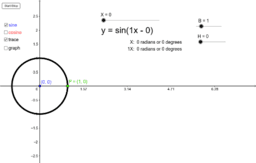•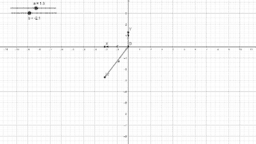Unit vectors, basis vectors and span

Activity

•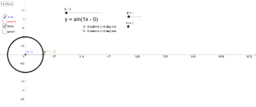Sine and cosine waves from the unit circle

Activity

•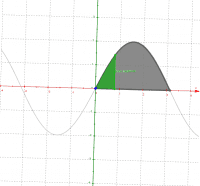y = 2 sin(x) square cross sections on 0 to pi

Activity

•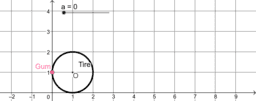gum on tire engage ny part 1

Activity

•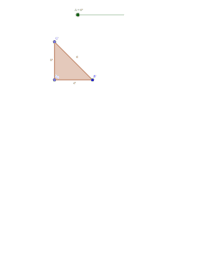Rotating a triangle around the y axis

Activity

•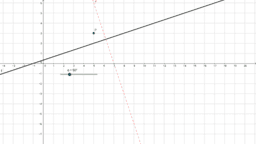Rotating a line around a point

Activity

•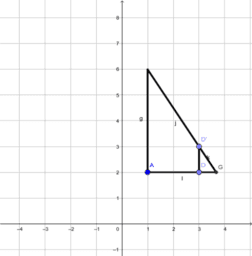Activity

•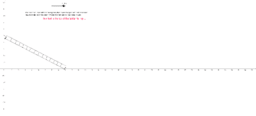Sliding ladder falling down related rates

Activity

•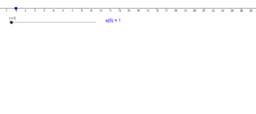Particle Moving Along a Line

Activity

•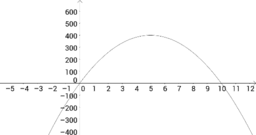y = 160x - 16x^2

Activity

•